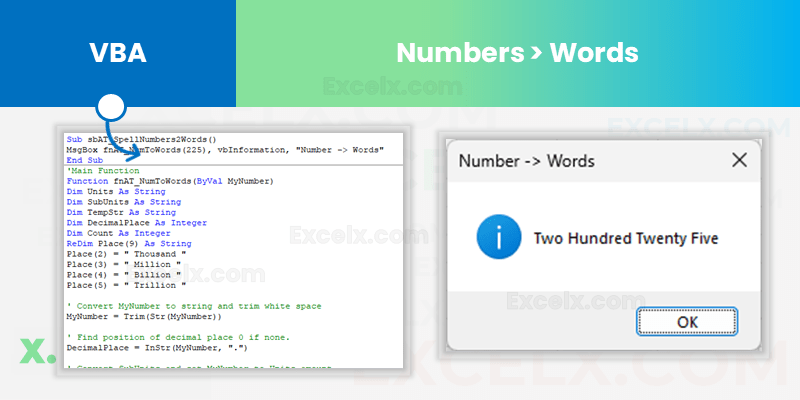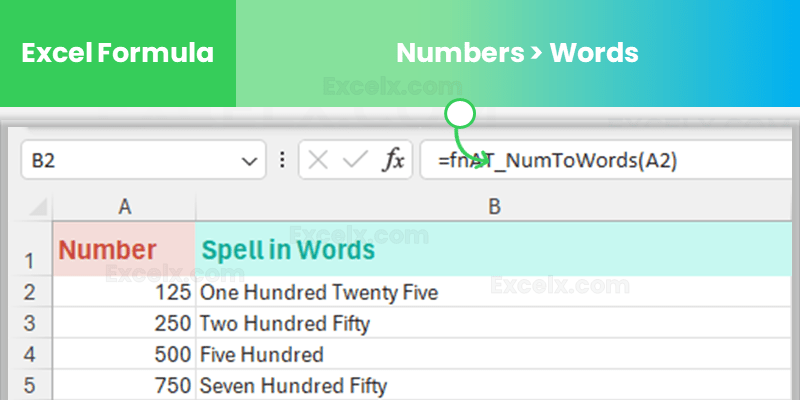Converting numbers to words in Excel is a common task, but Excel doesn’t have the built-in Function for this. However, it can be easily accomplished with the help of Visual Basic for Applications (VBA). In this blog post, we will walk you through the steps of creating a user-defined function that converts a numeric value to words.

We created Simple Excel Formula to Convert Numbers to Words. You can simply Copy and Paste the VBA Function and use it in both Excel Formulas and VBA Procedures. You can Pass any Number to the Function to Spell Number in Words.

## Steps to Convert Numbers to Words

Here are the simple steps for Converting numbers to words in Excel can be done by creating a custom function in Visual Basic for Applications (VBA).

1. Step 1: Open VBA Editor: To start the process, you will first need to open the VBA editor. This can be accomplished by pressing Alt + F11 on your keyboard while in Excel.
2. Step 2: Insert a New Module: Once the VBA editor is open, you should navigate to Insert > Module in the menu. This will create a new module where you can write your code.
3. Step 3: Write the Code/Copy Our NumToWords VBA Code: Within this new module, you will need to input the code that will create your custom function for converting numbers to words. You can either write your own code or use the VBA code provided in the previous section of this post.
4. Step 4: Use the Function: After the code is implemented, you can now use the new function just like any other built-in function in Excel. Simply return to your Excel sheet and in any cell, write =NumToWords(CELL). You should replace CELL with the cell number that contains the numeric value you wish to convert. For example, =NumToWords(A1) will convert the number in cell A1 to words.

With these steps, you can easily convert numbers to words in Excel, enhancing your data analysis and reporting capabilities.

## VBA to Convert Numbers to Words

Here is the easy to use Excel VBA Function to convert number to words. You can copy the below code and paste in your code module.```Option Explicit

'Main Function to Convert Number to Words
Function NumToWords(ByVal MyNumber)
Dim Units As String
Dim SubUnits As String
Dim TempStr As String
Dim DecimalPlace As Integer
Dim Count As Integer
ReDim Place(9) As String
Place(2) = " Thousand "
Place(3) = " Million "
Place(4) = " Billion "
Place(5) = " Trillion "

' Convert MyNumber to string and trim white space
MyNumber = Trim(Str(MyNumber))

' Find position of decimal place 0 if none.
DecimalPlace = InStr(MyNumber, ".")

' Convert SubUnits and set MyNumber to Units amount.
If DecimalPlace > 0 Then
SubUnits = GetTens(Left(Mid(MyNumber, DecimalPlace + 1) & "00", 2))
MyNumber = Trim(Left(MyNumber, DecimalPlace - 1))
End If

Count = 1
Do While MyNumber <> ""
TempStr = GetHundreds(Right(MyNumber, 3))
If TempStr <> "" Then Units = TempStr & Place(Count) & Units
If Len(MyNumber) > 3 Then
MyNumber = Left(MyNumber, Len(MyNumber) - 3)
Else
MyNumber = ""
End If
Count = Count + 1
Loop

NumToWords = Application.Trim(Units & SubUnits)
End Function

' Converts a number from 100-999 into text
Function GetHundreds(ByVal MyNumber)
Dim Result As String
If Val(MyNumber) = 0 Then Exit Function
MyNumber = Right("000" & MyNumber, 3)
' Convert the hundreds place.
If Mid(MyNumber, 1, 1) <> "0" Then
Result = GetDigit(Mid(MyNumber, 1, 1)) & " Hundred "
End If
' Convert the tens and ones place.
If Mid(MyNumber, 2, 1) <> "0" Then
Result = Result & GetTens(Mid(MyNumber, 2))
Else
Result = Result & GetDigit(Mid(MyNumber, 3))
End If
GetHundreds = Result
End Function

' Converts a number from 10 to 99 into text.
Function GetTens(TensText)
Dim Result As String
Result = "" ' Null out the temporary function value.
If Val(Left(TensText, 1)) = 1 Then ' If value between 10-19...
Select Case Val(TensText)
Case 10: Result = "Ten"
Case 11: Result = "Eleven"
Case 12: Result = "Twelve"
Case 13: Result = "Thirteen"
Case 14: Result = "Fourteen"
Case 15: Result = "Fifteen"
Case 16: Result = "Sixteen"
Case 17: Result = "Seventeen"
Case 18: Result = "Eighteen"
Case 19: Result = "Nineteen"
Case Else
End Select
Else ' If value between 20-99...
Select Case Val(Left(TensText, 1))
Case 2: Result = "Twenty "
Case 3: Result = "Thirty "
Case 4: Result = "Forty "
Case 5: Result = "Fifty "
Case 6: Result = "Sixty "
Case 7: Result = "Seventy "
Case 8: Result = "Eighty "
Case 9: Result = "Ninety "
Case Else
End Select
Result = Result & GetDigit(Right(TensText, 1)) ' Retrieve ones place.
End If
GetTens = Result
End Function

' Converts a number from 1 to 9 into text.
Function GetDigit(Digit)
Select Case Val(Digit)
Case 1: GetDigit = "One"
Case 2: GetDigit = "Two"
Case 3: GetDigit = "Three"
Case 4: GetDigit = "Four"
Case 5: GetDigit = "Five"
Case 6: GetDigit = "Six"
Case 7: GetDigit = "Seven"
Case 8: GetDigit = "Eight"
Case 9: GetDigit = "Nine"
Case Else: GetDigit = ""
End Select
End Function
```

Now that you have defined the function, you can use it just like any other Excel function. You can use it as Excel formula in a Cell or you can assign to a variable in VBA.

## Excel Formula to Convert Numbers to Words?

The `NumToWords` function, created in Excel VBA, converts numbers into their word equivalent. This is especially handy for writing checks, generating invoices, or creating certain reports. The example table provided uses this function. It has three columns: ‘Number’, ‘Formula’, and ‘Result’. ‘Number’ holds the numerical values. ‘Formula’ applies the `NumToWords` function to the numbers in Column A. ‘Result’ shows the output of the function – the verbal representation of the numbers.

To use this function, remember to first implement `NumToWords` in your Excel VBA as outlined in the prior steps.

`=NumToWords(A1)`Number(A) Formula(B) Result(C)
1 `=NumToWords(A2)` One
23 `=NumToWords(A3)` Twenty Three
456 `=NumToWords(A4)` Four Hundred Fifty Six
7890 `=NumToWords(A5)` Seven Thousand Eight Hundred Ninety
12345 `=NumToWords(A6)` Twelve Thousand Three Hundred Forty Five

Your number will be converted to words in the cell where you wrote the formula!

### Numbers to Words – VBA Use Cases

The `NumToWords` function in Excel VBA converts numeric values to words. To use it, you first open the VBA editor (`Alt + F11`), create a new module, and input the function code. Once it’s implemented, you can use it within your VBA scripts.

`=strNumberToWord=NumToWords(A1)`

For example:

1. Assign it to a variable:
• Dim result As String
• `result = NumToWords(123)`
2. Show the result in a message
• `MsgBox NumToWords(123)`
3. Enter the result in a cell,
• `Range("A1").Value = NumToWords(123)`
• which will enter the converted word form of the number into cell A1. This function extends Excel’s capabilities and offers more flexibility.

Remember, user-defined functions in Excel VBA are powerful and flexible tools that allow you to create new functionality, such as converting numbers to words. However, they are a bit more complex and require knowledge of VBA to create and debug.

Here is the Example file with Excel formula to Convert Number to Words. You can see the Example of Function using in both Excel Formula and VBA.

Convert Numbers to Words in Excel

### Conclusion

Note: Keep in mind that this VBA function will only work with whole numbers between 0 and 999999999999999. If you need to work with very large numbers or decimals, the code would need to be modified.

We hope you found our post on converting numbers to words in Excel valuable and practical. Your thoughts and feedback are extremely important to us!

If you’ve tried this technique or have any questions about it, please feel free to share your experiences in the comments below. We’d love to hear how this function has worked for you or if there are any other methods you’ve found useful.

Your input helps us make our content better and more relevant to your needs. Additionally, if you’ve encountered any issues or difficulties along the way, share those as well so we can improve our instructions.

Thank you again for reading our blog. Your engagement helps us create more useful content for you and other readers.